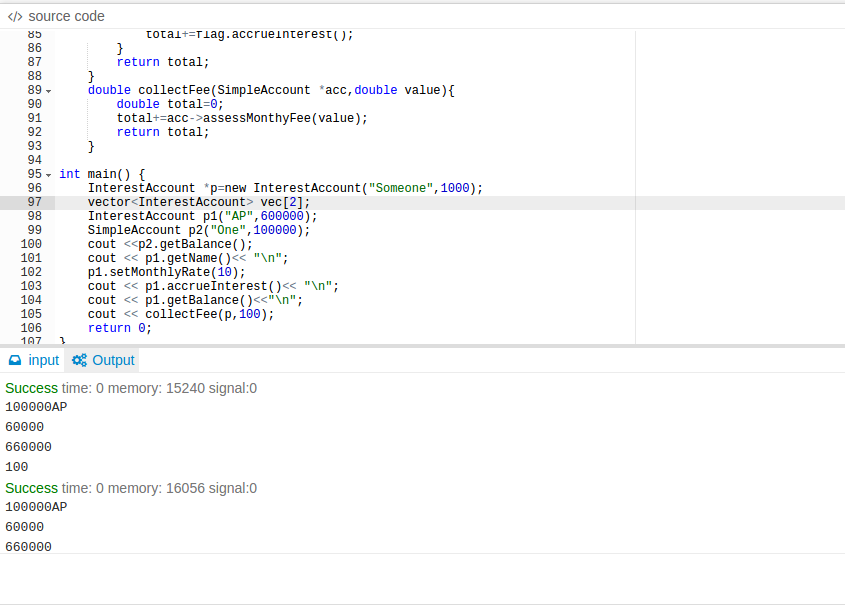C++ Assignment

Don't use plagiarized sources. Get Your Custom Essay on
GET AN ESSAY WRITTEN FOR YOU FROM AS LOW AS \$13/PAGE

Here is the question.

You are writing some simple code to model operations in a bank. For simplicity, all operations are in pennies. The bank has two different types of accounts: simple accounts and interest accounts.

A SimpleAccount has these behaviors:

create an account, with the given customer name and with the initial deposit amount

deposit – deposit some amount and return new balance

withdraw – withdraw some amount and return amount withdrawn. If insufficient balance, whole balance is withdrawn.

assessMonthlyFee – reduce balance by the given fee amount and return the fee collected. If insufficient balance, whole balance is collected.

getBalance – return the current balance

getName – return the account owner

An InterestAccount is like a simple account but the rules are a bit different. The monthly fee is waived if the balance is 100000 or more and the account accrues interest at the current monthly interest rate. The account has these new behaviors:

setMonthlyRate – set the monthly interest rate to the given rate

accrueInterest – add the accrued interest to the current balance and return the interest paid. E.g.: if rate is 2% and the balance is 200, the balance becomes 204.

a) Please give the interface and implementation of the SimpleAccount and InterestAccount classes.

b) Please implement a function named payInterest that takes a vector of InterestAccounts and pays the monthly accrual of interest to each account. The function returns the total interest paid.

c) Please implement a function named collectFee that takes a pointer to an account and a fee amount and assesses the given monthly fee on that account. It returns the fee collected. The function should work correctly for both types of accounts.

(a)

class Bank{ //interface is nothing but a set of virtual functions
virtual double deposit(double value)=0; //common to both the types of account
virtual double withdraw(double value)=0;
virtual double getBalance()=0;
virtual double assessMonthyFee(double value)=0;
virtual string getName()=0; //pure virtual functions
};
class SimpleAccount:public Bank{ //implementing the interface
protected:
string name; //have protected name and amount which can be inherited
double amount; //by InterectAccount class
public:

SimpleAccount(string str, double value) {
name=str; //contructor to set the name and amount
amount=value;
}
double deposit(double value) {
amount += value; //implementation of all the functions
return amount;
}
double withdraw(double value) {
if (amount < value ) {
amount=0; //if withdraw is less than value, withdraw all
return amount;
}
amount-=value;
return value; //return withdrawn money
}
double getBalance(){
return amount; //return amount
}
double assessMonthyFee(double value){
if (amount < value ) {
amount=0; //if value is greater than amount
return amount; //deduct all and return deducted
}
amount-=value;
return value;
}
string getName(){
return name; //get name of the holder
}
};

class InterestAccount:public SimpleAccount{ //inherits the simple account
private:
double rate; //rate variable as this is interest account
public:
InterestAccount(string name, double amount):SimpleAccount(name, amount) {

}
double assessMonthyFee(double value){
if(amount >= 100000){ //has a special feature if amount is greater
return 0; //than 100000 no deduction
}
if (amount < value ) {
return amount;
}
amount-=value;
return value;
}
void setMonthlyRate(double rate1){
rate=rate1; //set rate to the given rate
}
double accrueInterest(){
}
};

(b)    double payInterest(vector<InterestAccount> vec){
double total=0; //takes vector and iterates for all its members
for(InterestAccount flag : vec){
total+=flag.accrueInterest(); //adding the total to previous total
}
Here I am attaching a sample output for your better understanding: